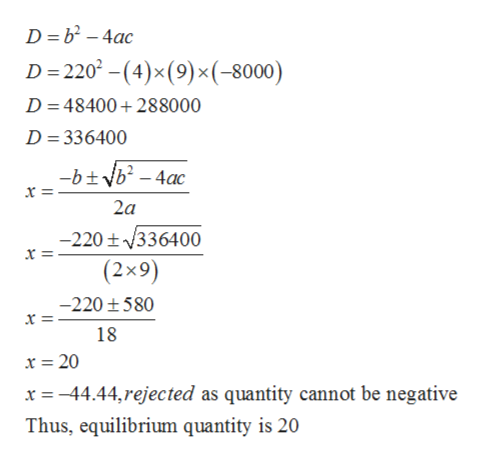# Consider a demand and supply scenario for 3-D glasses. An independent contracting firm was so kind and estimated the supply curve as a function of the quantity x as s(x)=.06x2 + 1.8 x + 60 and the demand as d(x)= -.075 x2 – 1.5x +180.a) Solve for the equilibrium quantity and price. What condition has to hold in an equilibrium? For full credit you must show ALL steps of your algebra.b) Graph the supply and demand curve. Clearly mark the proper price and quantity intercept. Show how you calculated the intercepts.

Question
62 views

Consider a demand and supply scenario for 3-D glasses. An independent contracting firm was so kind and estimated the supply curve as a function of the quantity x as s(x)=.06x2 + 1.8 x + 60 and the demand as d(x)= -.075 x2 – 1.5x +180.

a) Solve for the equilibrium quantity and price. What condition has to hold in an equilibrium? For full credit you must show ALL steps of your algebra.

b) Graph the supply and demand curve. Clearly mark the proper price and quantity intercept. Show how you calculated the intercepts.

check_circle

Step 1

(a)

The combination of demand and supply forces determine what amount of goods to be produced and consumed in an economy and at what price. These stable values of price and quantity are known as equilibrium price and equilibrium quantity respectively.

Given

Step 2

For economy to be in equilibrium, demand and supply forces must be equal. Thus, according to this necessary condition of equilibrium, the intersection point of demand and supply curves determine equilibrium price and equilibrium quantity.

Thus, for equilibrium price and quantity

Equate given supply and demand equations

Step 3

Using the discrimin...help_outlineImage TranscriptioncloseD b2-4ac D 220 (4)x(9)x(-8000) D 48400288000 D 336400 - -btvb-4ac x = 2a -220t336400 (2x9) -220 580 x = 18 x 20 x 44.44,rejected as quantity cannot be negative Thus, equilibrium quantity is 20 fullscreen

### Want to see the full answer?

See Solution

#### Want to see this answer and more?

Solutions are written by subject experts who are available 24/7. Questions are typically answered within 1 hour.*

See Solution
*Response times may vary by subject and question.
Tagged in